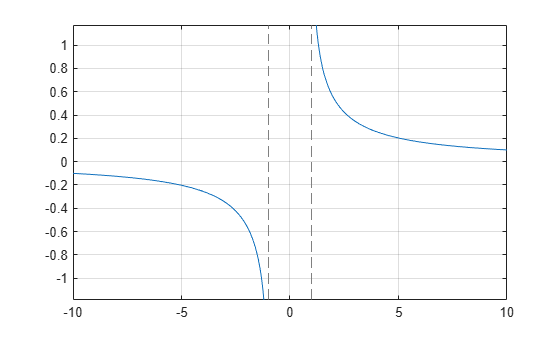# acoth

Symbolic inverse hyperbolic cotangent function

## Syntax

``acoth(X)``

## Description

example

````acoth(X)` returns the inverse hyperbolic cotangent function of `X`.```

## Examples

### Inverse Hyperbolic Cotangent Function for Numeric and Symbolic Arguments

Depending on its arguments, `acoth` returns floating-point or exact symbolic results.

Compute the inverse hyperbolic cotangent function for these numbers. Because these numbers are not symbolic objects, `acoth` returns floating-point results.

`A = acoth([-pi/2, -1, 0, 1/2, 1, pi/2])`
```A = -0.7525 + 0.0000i -Inf + 0.0000i 0.0000 + 1.5708i... 0.5493 + 1.5708i Inf + 0.0000i 0.7525 + 0.0000i```

Compute the inverse hyperbolic cotangent function for the numbers converted to symbolic objects. For many symbolic (exact) numbers, `acoth` returns unresolved symbolic calls.

`symA = acoth(sym([-pi/2, -1, 0, 1/2, 1, pi/2]))`
```symA = [ -acoth(pi/2), Inf, -(pi*1i)/2, acoth(1/2), Inf, acoth(pi/2)]```

Use `vpa` to approximate symbolic results with floating-point numbers:

`vpa(symA)`
```ans = [ -0.75246926714192715916204347800251,... Inf,... -1.5707963267948966192313216916398i,... 0.54930614433405484569762261846126... - 1.5707963267948966192313216916398i,... Inf,... 0.75246926714192715916204347800251]```

### Plot Inverse Hyperbolic Cotangent Function

Plot the inverse hyperbolic cotangent function on the interval from -10 to 10.

```syms x fplot(acoth(x),[-10 10]) grid on```### Handle Expressions Containing Inverse Hyperbolic Cotangent Function

Many functions, such as `diff`, `int`, `taylor`, and `rewrite`, can handle expressions containing `acoth`.

Find the first and second derivatives of the inverse hyperbolic cotangent function:

```syms x diff(acoth(x), x) diff(acoth(x), x, x)```
```ans = -1/(x^2 - 1) ans = (2*x)/(x^2 - 1)^2```

Find the indefinite integral of the inverse hyperbolic cotangent function:

`int(acoth(x), x)`
```ans = log(x^2 - 1)/2 + x*acoth(x)```

Find the Taylor series expansion of `acoth(x)` for ```x > 0```:

```assume(x > 0) taylor(acoth(x), x)```
```ans = x^5/5 + x^3/3 + x - (pi*1i)/2```

For further computations, clear the assumption on `x` by recreating it using `syms`:

`syms x`

Rewrite the inverse hyperbolic cotangent function in terms of the natural logarithm:

`rewrite(acoth(x), 'log')`
```ans = log(1/x + 1)/2 - log(1 - 1/x)/2```

## Input Arguments

collapse all

Input, specified as a symbolic number, variable, expression, or function, or as a vector or matrix of symbolic numbers, variables, expressions, or functions.

## Version History

Introduced before R2006a